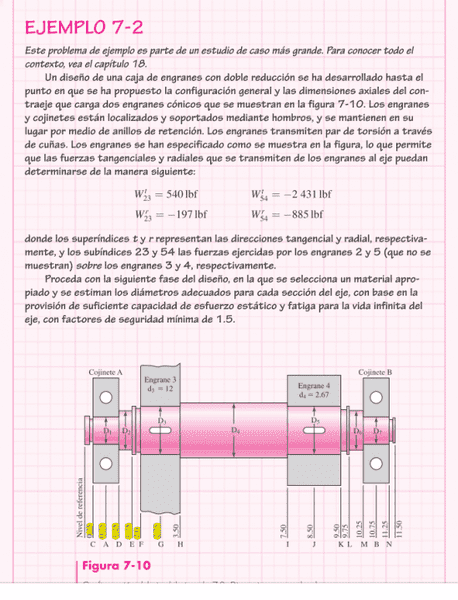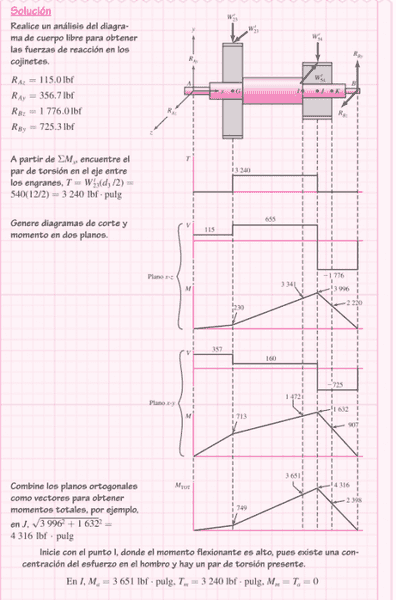# Bending moment in an axis doesn´t match

Hey guys, first of all, this isn't a homework question, I just want to know why the results are what they are. I've been trying t obtain the same numbers using moment equilibrium but they still don't match. This is the example:W are the forces applied by the gears in force-pounds. The gears are located at the sides of the thickest part of the axle which is the diameter D4. The distances in inches are located below where the letters are.To obtain the graphs analysing x-y axis and z-x axis is necessary. The reactions in the bearings are Raz, Ray, Rbz and Rby. These are used to obtain the shear force diagrams and the bending moment diagrams. I'm unable to obtain the values in the bending moment diagrams. Also, if you could tell me why the torsion diagram (the first one above with the number 3240 on it) says torque is constant despite the change in the circular section I would really appreciate it.
Thank you guys!